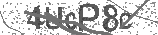﻿ The 4 Times Table Song (Multiplying by 4) | Silly School Songs | Safe Videos for Kids
Welcome

# The 4 Times Table Song (Multiplying by 4) | Silly School Songs

Thanks! Share it with your friends!

URL

You disliked this video. Thanks for the feedback!

Sorry, only registred users can create playlists.URLChannel: Silly School Songs
Categories: Basic Math   |   MathFind Related Videos  added
72 Views## Description

INTERACTIVE LEARNING - FLAShCARDS CLICK below!
http://sillyschoolsongs.com/lessonplans/multiplyby4

Learn how to multiply by 4 with this fun and catchy 4 times table song for kids. For more information and music, visit http://sillyschoolsongs.com.

It's time to multiply by 4
It's time to multiply by 4
It's time to multiply by 4
"Well go ahead and do it, whatcha waitin' for?"

4 x 1 = 4
4 x 2 = 8
4 x 3 = 12
4 x 4 = 16
4 x 5 = 20
4 x 6 = 24
4 x 7 = 28
4 x 8 = 32
4 x 9 = 36
4 x 10 = 40
4 x 11 = 44
4 x 12 = 48

Excellent job everyone!
Now we're going to turn the numbers around and try it again,
Starting with 1 x 4 and going up. Ready? Here we go!

1 x 4 = 4
2 x 4 = 8
3 x 4 = 12
4 x 4 = 16
5 x 4 = 20
6 x 4 = 24
7 x 4 = 28
8 x 4 = 32
9 x 4 = 36
10 x 4 = 40
11 x 4 = 44
12 x 4 = 48

See, that wasn't so hard, was it?
Now you know how to multiply by 4.
Just keep practicing, and we'll see you later!Be the first to comment